Next: Boundary conditions on the Up: Electrostatics Previous: Ohm's law

## Conductors

Most (but not all) electrical conductors obey Ohm's law. Such conductors are termed ohmic. Suppose that we apply an electric field to an ohmic conductor. What is going to happen? According to Eq. (613), the electric field drives currents. These redistribute the charge inside the conductor until the original electric field is canceled out. At this point, the currents stop flowing. It might be objected that the currents could keep flowing in closed loops. According to Ohm's law, this would require a non-zero e.m.f.,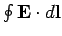, acting around each loop (unless the conductor is a superconductor, with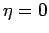). However, we know that in steady-state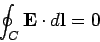(617)

around any closed loop. This proves that a steady-state e.m.f. acting around a closed loop inside a conductor is impossible. The only other alternative is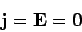(618)

inside a conductor. It immediately follows from the Maxwell equation,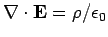, that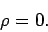(619)

So, there are no electric charges in the interior of a conductor. But, how can a conductor cancel out an applied electric field if it contains no charges? The answer is that all of the charges reside on the surface of the conductor. In reality, the charges lie within one or two atomic layers of the surface (see any textbook on solid-state physics). The difference in scalar potential between two points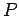and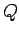is simply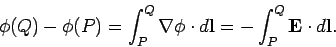(620)

However, ifandlie inside the same conductor then it is clear from Eq. (620) that the potential difference betweenandis zero. This is true no matter whereandare situated inside the conductor, so we conclude that the scalar potential must be uniform inside a conductor. A corollary of this is that the surface of a conductor is an equipotential (i.e.,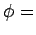constant) surface.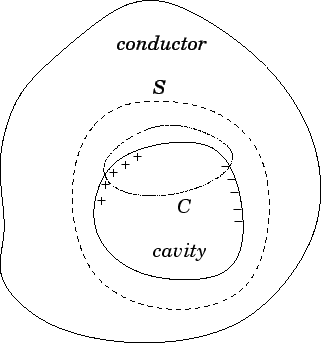Not only is the electric field inside a conductor zero. It is also possible to demonstrate that the field within an empty cavity lying inside a conductor is also zero, provided that there are no charges within the cavity. Let us, first of all, apply Gauss' law to a surfacewhich surrounds the cavity, but lies wholly in the conducting material (see Fig. 41). Since the electric field is zero in a conductor, it follows that zero net charge is enclosed by. This does not preclude the possibility that there are equal amounts of positive and negative charges distributed on the inner surface of the conductor. However, we can easily rule out this possibility using the steady-state relation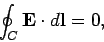(621)

for any closed loop. If there are any electric field-lines inside the cavity then they must run from the positive to the negative surface charges. Consider a loopwhich straddles the cavity and the conductor, such as the one shown in Fig. 41. In the presence of field-lines, it is clear that the line integral of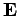along that portion of the loop which lies inside the cavity is non-zero. However, the line integral ofalong that portion of the loop which runs through the conducting material is obviously zero (since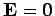inside a conductor). Thus, the line integral of the field around the closed loopis non-zero. This, clearly contradicts Eq. (621). In fact, this equation implies that the line integral of the electric field along any path which runs through the cavity, from one point on the interior surface of the conductor to another, is zero. This can only be the case if the electric field itself is zero everywhere inside the cavity. There is one proviso to this argument. The electric field inside a cavity is only zero if the cavity contains no charges. If the cavity contains charges then our argument fails because it is possible to envisage that the line integral of the electric field along many different paths across the cavity could be zero without the fields along these paths necessarily being zero (this argument is somewhat inexact: we shall improve it later on).

We have shown that if a cavity is completely enclosed by a conductor then no stationary distribution of charges outside can ever produce any fields inside. So, we can shield a piece of electrical equipment from stray external electric fields by placing it inside a metal can. Using similar arguments to those given above, we can also show that no static distribution of charges inside a closed conductor can ever produce a field outside the conductor. Clearly, shielding works both ways!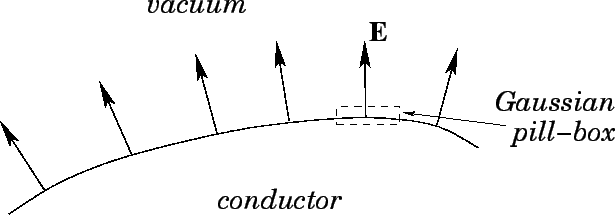Let us consider some small region on the surface of a conductor. Suppose that the local surface charge density is, and that the electric field just outside the conductor is. Note that this field must be directed normal to the surface of the conductor. Any parallel component would be shorted out by surface currents. Another way of saying this is that the surface of a conductor is an equipotential surface. We know that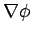is always perpendicular to equipotential surfaces, so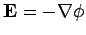must be locally perpendicular to a conducting surface. Let us use Gauss' law,(622)

whereis a so-called Gaussian pill-box (see Fig. 42). This is a pill-box shaped volume whose two ends are aligned normal to the surface of the conductor, with the surface running between them, and whose sides are tangential to the surface normal. It is clear thatis perpendicular to the sides of the box, so the sides make no contribution to the surface integral. The end of the box which lies inside the conductor also makes no contribution, sinceinside a conductor. Thus, the only non-zero contribution to the surface integral comes from the end lying in free space. This contribution is simply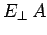, where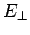denotes an outward pointing (from the conductor) normal electric field, andis the cross-sectional area of the box. The charge enclosed by the box is simply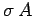, from the definition of a surface charge density. Thus, Gauss' law yields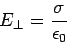(623)

as the relationship between the normal electric field immediately outside a conductor and the surface charge density.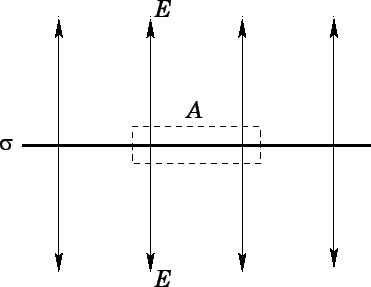Let us look at the electric field generated by a sheet charge distribution a little more carefully. Suppose that the charge per unit area is. By symmetry, we expect the field generated below the sheet to be the mirror image of that above the sheet (at least, locally). Thus, if we integrate Gauss' law over a pill-box of cross sectional area, as shown in Fig. 43, then the two ends both contribute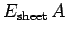to the surface integral, where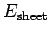is the normal electric field generated above and below the sheet. The charge enclosed by the pill-box is just. Thus, Gauss' law yields a symmetric electric field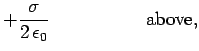(624)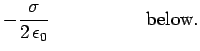(625)

So, how do we get the asymmetric electric field of a conducting surface, which is zero immediately below the surface (i.e., inside the conductor) and non-zero immediately above it? Clearly, we have to add in an external field (i.e., a field which is not generated locally by the sheet charge). The requisite field is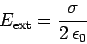(626)

both above and below the charge sheet. The total field is the sum of the field generated locally by the charge sheet and the external field. Thus, we obtain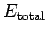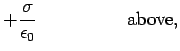(627)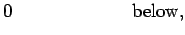(628)

which is in agreement with Eq. (623).

The external field exerts a force on the charge sheet. The field generated locally by the sheet itself obviously cannot exert a force (the sheet cannot exert a force on itself!). The force per unit area acting on the surface of the conductor always acts outward, and is given by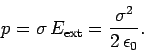(629)

Thus, there is an electrostatic pressure acting on any charged conductor. This effect can be visualized by charging up soap bubbles: the additional electrostatic pressure eventually causes them to burst. The electrostatic pressure can also be written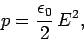(630)

whereis the field strength immediately above the surface of the conductor. Note that, according to the above formula, the electrostatic pressure is equivalent to the energy density of the electric field immediately outside the conductor. This is not a coincidence. Suppose that the conductor expands by an average distance, due to the electrostatic pressure. The electric field is excluded from the region into which the conductor expands. The volume of this region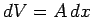, whereis the surface area of the conductor. Thus, the energy of the electric field decreases by an amount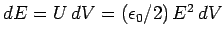, where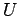is the energy density of the field. This decrease in energy can be ascribed to the work which the field does on the conductor in order to make it expand. This work is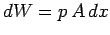, where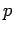is the force per unit area the field exerts on the conductor. Thus,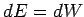, from energy conservation, giving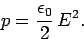(631)

This technique for calculating a force given an expression for the energy of a system as a function of some adjustable parameter is called the principle of virtual work, and is very useful.

We have seen that an electric field is excluded from the inside of the conductor, but not from the outside, giving rise to a net outward force. We can account for this by saying that the field exerts a negative pressureon the conductor. We know that if we evacuate a metal can then the pressure difference between the inside and the outside eventually causes it to implode. Likewise, if we place the can in a strong electric field then the pressure difference between the inside and the outside will eventually cause it to explode. How big a field do we need before the electrostatic pressure difference is the same as that obtained by evacuating the can? In other words, what field exerts a negative pressure of one atmosphere (i.e.,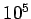newtons per meter squared) on conductors? The answer is a field of strength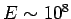volts per meter. Fortunately, this is a rather large field, so there is no danger of your car exploding when you turn on the stereo!Next: Boundary conditions on the Up: Electrostatics Previous: Ohm's law
Richard Fitzpatrick 2006-02-02